Interesting script?
So post a link to it -
let others appraise it
You liked the script? Try it in the MetaTrader 5 terminal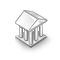# Fuzzy - library for developing fuzzy models - library for MetaTrader 5

Дмитрий Калюжный |
Views:
9960
Rating:
(34)
Published:
2015.08.27 11:31
Updated:
2017.05.19 18:12

Real Author

Dmitry Kalyuzhny. FuzzyNet project website - http://sourceforge.net/projects/fuzzynet/

Unzip the archive into the terminal_data_folder.
The library codes are located in the <terminal_data_folder>\MQL5\Include\Math\FuzzyNet\
Sample test scripts can be found in the <terminal_data_folder>\MQL5\Scripts\FuzzyNet\

FuzzyNet is one of the most popular mathematical libraries for creating fuzzy models

Fuzzy Logic Library for Microsoft.Net (FuzzyNet) is an easy to use component that implements Mamdani and Sugeno fuzzy inference systems.

FuzzyNet includes:

The following additions have been made when converting the library into MQL5:

• 8 new membership functions.
• 4 new defuzzification methods for Mamdani-type systems.

Using the Library

1. Depending on your task, create an empty fuzzy Mamdani or Sugeno-type system.
2. Create fuzzy input and output variables.
3. Appropriate terms are added to each fuzzy variable. Before that, a name and a membership function are assigned to a term.
4. Variables are added to the system.
5. A set of rules is created.
6. The rules are added to the system.
7. Input data is sent to the system.
8. The system calculation function is called.

Note: The Mamdani-type inference system can be configured at any stage after its creation before the system calculation function is called. If the system settings have not been changed after its creation, the system works with default settings:

• Implication is performed using the Min operator (an output fuzzy set is truncated).
• Aggregation is performed using the Max operator (receiving maximum values of the output membership functions obtained after implication).
• Defuzzification is performed using the center of gravity method.

Conversion of the FuzzyNet library (v. 1.2.0) is displayed below.

To work with the library, include MamdaniFuzzySystem.mqh or SugenoFuzzySystem.mqh file depending on the system you are creating.

Below is more detailed information about FuzzyNet ported library packages:
Packages
Description
Dictionary.mqh
The package contains additional classes necessary for other packages.
FuzzyRule.mqh
Classes for creating fuzzy rules:
1. GenericFuzzyRule - class for creating a non-fuzzy rule condition.
2. MamdaniFuzzyRule - class for creating a Mamdani-type fuzzy rule conclusion.
3. SugenoFuzzyRule - class for creating a Sugeno-type fuzzy rule conclusion.

The package also contains auxiliary classes for implementing fuzzy rules.

FuzzyTerm.mqh Package for creating fuzzy terms.
FuzzyVariable.mqh Package for creating fuzzy variables.
GenericFuzzySystem.mqh The class implements the common functionality for Mamdani and Sugeno systems.
Helper.mqh The package contains additional classes necessary for other packages.
InferenceMethod.mqh The package contains additional classes necessary for other packages.
MamdaniFuzzySystem.mqh The class for creating a Mamdani-type fuzzy system.
MembershipFunction.mqh Classes of membership functions:
1. Gaussian membership function.
2. Two-sided Gaussian membership function.
3. Generalized bell-shaped membership function.
4. S-shaped membership function.
5. Z-shaped membership function.
6. Pi-shaped membership function.
7. Sigmoid membership function.
8. Product of two sigmoid membership functions.
9. Membership function in the form of a difference between two sigmoid functions.
10. Trapezoidal membership function.
11. Triangular membership function.
12. Membership function in the form of a constant.
13. Membership function as a composition of membership functions.
RuleParser.mqh Class for analyzing fuzzy rules.
SugenoFuzzySystem.mqh Class for creating a Sugeno-type fuzzy system.
SugenoVariable.mqh The package contains the following classes:
1. LinearSugenoFuction - class for creating linear functions.
2. SugenoVariable - class for creating a Sugeno-type fuzzy variable.

Sugeno-type fuzzy variables are used when developing rules for a Sugeno-type system.

Using FuzzyNet Library in MQL5

Before writing a fuzzy system, you should have a clear vision of its elements, including:

1. Number of input and output values to be rearranged by fuzzy variables.
2. Number of terms and their membership functions for each fuzzy variable.
4. Number and content of fuzzy rules corresponding to the selected system.
5. Specific parameters and features of the system.

The system development and calculation:

1. Create an empty system.

For a Mamdani-type system:

`MamdaniFuzzySystem *fuzzy_system=new MamdaniFuzzySystem();`
For a Sugeno-type system:
`SugenoFuzzySystem *fuzzy_system=new SugenoFuzzySystem();`
2. Create all fuzzy input variables separately from the system by assigning a name and maximum/minimum values to all variables as parameters:
`FuzzyVariable *fuzzy_variable=new FuzzyVariable(const string name,const double min,const double max);`
3. Create functions of fuzzy terms, create fuzzy terms themselves and pass appropriate names and membership functions to them. After that, add the terms to the corresponding variables. To reduce the program code, the process can be written as follows:
`fuzzy_variable.Terms().Add(new FuzzyTerm(const string name,new IMembershipFunction());`
4. Enter the input variables to the system:
`fuzzy_system.Input().Add(FuzzyVariable fuzzy_variable);`
5. Create input variables paying attention to your system type. For a Mamdani-type system, creation is similar to steps 2 and 3. For a Sugeno-type model, create special fuzzy variables accepting only a variable name as parameters:
`SugenoVariable *sugeno_variable=new SugenoVariable(const string name);`
Linear functions interpreting the linear combination of input values are added to a Sugeno-type fuzzy variable instead of fuzzy terms. A name and a coefficient array are used as linear function parameters. A linear equation is formed based on that array, therefore, it is important to comply with the order of elements in the array. A coefficient array length should be equal to the amount of input values or exceed it by one. If the lengths are equal, an absolute term of an equation is equal to zero. If the array length exceeds the amount by one, an absolute term is equal to the last element value. All other array elements beginning from the first one are assigned to fuzzy input variables in the order they were entered into the system.
`sugeno_varriable.Functions().Add(fuzzy_sytem.CreateSugenoFunction(const string name, const double &coeffs[]));`
6. Similar to the step 4, output variables should also be added to the system:

For a Mamdani-type system:

`fuzzy_system.Output().Add(FuzzyVariable fuzzy_variable);`

For a Sugeno-type system:

`fuzzy_system.Output().Add(FuzzyVariable fuzzy_variable);`
7. Arrange a set of rules according to the system. The rules are defined as a common string and automatically analyzed based on keywords. The keywords are "if", "then", "is", "and", "or", "not", "(" , ")", "slightly", "somewhat", "very" and "extremely", as well as all names of variables, terms and functions available in your system.

For a Mamdani-type system:

`MamdaniFuzzyRule *fuzzy_rule = fuzzy_system.ParseRule(const string rule_text);`

For a Sugeno-type system:

`SugenoFuzzyRule *fuzzy_rule = fuzzy_system.ParseRule(const string rule_text);`
8. Enter all rules into the system:

For a Mamdani-type system:

`fuzzy_system.Rules().Add(MamdaniFuzzyRule fuzzy_rule);`

For a Sugeno-type system:

`fuzzy_system.Rules().Add(SugenoFuzzyRule fuzzy_rule);`
9. Pass input values of the variables to the system for calculation. To do this, they should be defined. At its input, the system accepts the list of values including the Dictionary_Obj_Double class object. The class is described in the Dictionary.mqh file.
`Dictionary_Obj_Double *p_od_in=new Dictionary_Obj_Double;`
The class implements the SetAll(CObject *key, const double value) method accepting two parameters - a fuzzy variable and a numerical value. This element is an input variable of the system.
`p_od_in.SetAll(FuzzyVariable fuzzy_variable,const double value);`
All other input values are filled the same way. Create the list and add all values to it:
```CList *in=new CList;
10. The output values should be specified as well:
```Dictionary_Obj_Double *p_od_out=new Dictionary_Obj_Double;
CList *out=new CList;```
11. Call the Calculate(CList *&list) function for our system returning the list of the system calculation results:
`out=fuzzy_system.Calculate(in);`
After that, the out list stores all calculated output values in the order they were entered into the system. We only need to receive them:
```p_od_out=out.GetNodeAtIndex(int index);
double result=p_od_out.Value();```
Now, the result variable stores the system calculation result for an output value entered into the system under a number specified in index.

Sample Scripts

Tips Sample (Mamdani)

Tips_Sample_Mamdani.mq5 calculates the tip percentage you need to pay depending on the quality of service and food.

Enter the input parameters: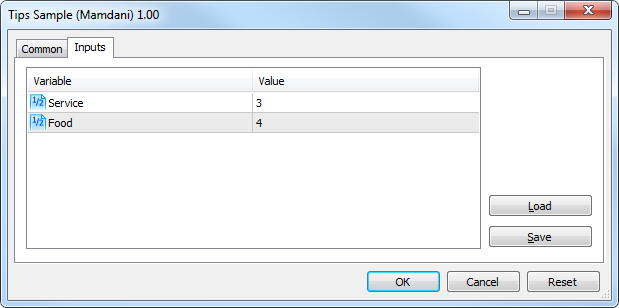Calculation results: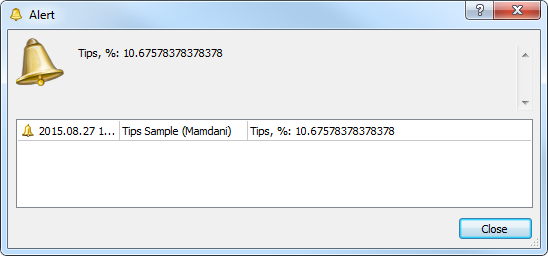Cruise Control Sample (Sugeno)

Cruise_Control_Sample_Sugeno.mq5 sample script is an example of a fuzzy regulator. It represents a car cruise control system that calculates the necessary acceleration using the data on the current deviation and the deviation rate of change in order for the car to reach a desired speed.

Enter the input parameters:

###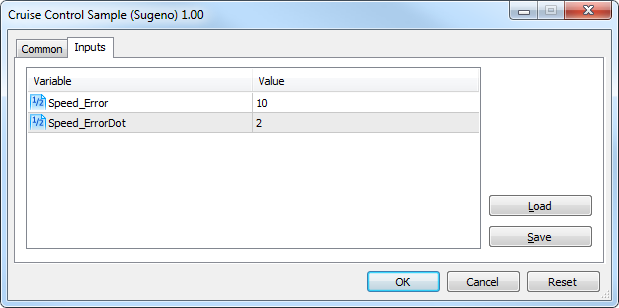Calculation results: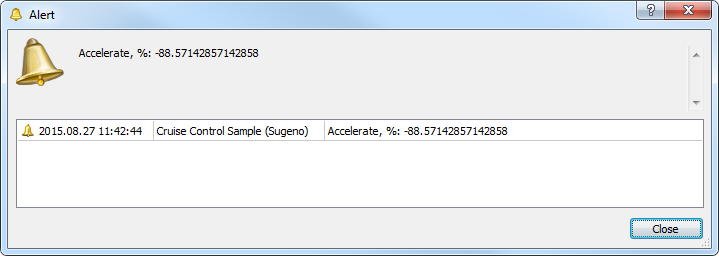Translated from Russian by MetaQuotes Ltd.
Original code: https://www.mql5.com/ru/code/13697CWndContainer Replacement

This file replaces the WndContainer.mqh original file. It prevents the chart from being affected when you click and hold the mouse inside a container area.DonchianChannels_HTF

The DonchianChannels indicator with the timeframe selection option available in the input parameters.CRect and CCanvas replacement (Standard Library - several bugs fixed)

Bug fixed versions of CRect and CCanvas which are part of the standard library.Flat-Trend_HTF

The Flat-Trend indicator with the timeframe selection option available in the input parameters.